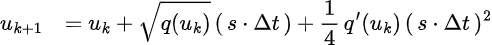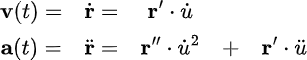# Online Feedrate Override

Does the planning algorithm support online change of the feedrate? For example, the user can enlarge of lower the maximum feedrate at runtime. The optimization should re-run to respect the new feedrate constraint.

Yes, OpenCN supports online change of the feedrate, often called by CNC manufacturers “Override”.

The Override is acting at the “resampling” phase after the feedrate optimization.
It’s simply a scale factor on the time axis.
Therefore, it not only changes the maximum feedrate, but also the acceleration and jerk limits.
Everything is slowed down or speeded up.

At the moment being, the resampling takes place in the user space, and there is a FIFO buffer of setpoint values between user and kernel space.

This buffer induces a loss of reactivity when changing the feedrate.

We decided as a low priority task to move the resampling in the kernel space in order to avoid the time lag created by the FIFO buffer.

Hi Raoul, Do you mean the following resampling function:
`function state = ResampleNoCtx(state, Bl, Curv) `
But I didn’t see a time scaling factor in the input interface.

Hi Yakunix,

There are two ways to change the feedrate in our algorithm:

• The federate is passed directly in our curvStruct. This value is then used to define the matrix of constraints of the optimization problem ( Have a look to the function `buildConstr` in FeedratePlanning_LP) )
• In the C++ implementation, we used a parameter called Override that can be changed from the user GUI. This parameter will directly rescale the time step used during the resampling. A smaller value of time step will reduce the federate. Note, the rate used to send the commands to the drive remains the same. ( See Class LowPass` ` : feedopt.cpp ) This code isn’t present in opencn-matlab, it is native C/C++ code.

Best

I see. So a low pass filter is used to smoothly change the sampling period. Should the velocity and acceleration also be scaled when doing the evaluation? Or we just use the position command without vel/acc send to the servor?

Only position setpoint values are sent via EtherCAT to the drives.
This is the EtherCAT standard (CSP mode).

The drives recovers from the received position data the velocity and acceleration for feedforward purposes

Hi,
Adding a scaling to the time step used during the resampling changes the equation (7) from the publication openCN :If the LP optimization leaded to a feasible solution, both velocity and acceleration constraints are ensured to be satisfied for given time step ( scaling = 1 ).

In the particular case where s is smaller than 1 but bigger than 0 ( => a lower feedrate than before we will be optained ), both velocity and acceleration should remain bellow the constraints used during the optimization since we have :If the scaling factor is bigger than 1, the resulting trajectory might violate the constraints.

To resume, I think the time scaling used during resampling rescales both speed and acceleration even if only the position is sent to the drive.

best,

1 Like

If Override = 0.5, the max velocity is diveded by 2, the max acceleration is divided by 4, and the max jerk is divided by 8.

This is simply a consequence of the time scaling.

In the function ResampleNoCtx, there is a remaining time estimation:

If the dt is changed online, will the Trest still be valid?

For example if scaling = 0.5, the remaining time should be 2 * Trest?

dear yakunix,

It’s the end of semester here and I’m extremely busy with exams and correctionsThanks Raoul. Take your time!